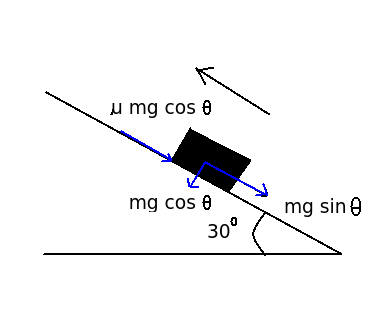# Numerical - Distance traveled by a block upwards on an inclined plane

Q.  What is the maximum distance traveled by a block moving upwards on an inclined plane of 30o with velocity of 20 m/s, if coefficient of friction is 0.23 between the block and inclined plane?
- Published on 18 Sep 15

a. 29.19 m
b. 22.56 m
c. 17.32 m
d. 17.00 m

#### Discussion

• Sravanthi   -Posted on 15 Dec 15
Given: Velocity of block = 20 m/s, coefficient of friction = 0.23

Formula: Force = mass x acceleration

Solution:1) Force = mass x acceleration

mg sin θ – μmg cos θ = ma

Substituting the given values, we get

a = - ( g sin θ + μg cos θ)

a = - ( 9.81 x sin 30 + 0.23 x 9.81 x cos 30)

a = - (4.905 + 1.954)

a = - 6.859

2) Using kinematic equation, v2 = u2 + 2as

Substituting the value of a, we get

0 = (20)2 + [2 x (-6.89) x s]

s = 29.19 m

The maximum distance traveled by a block moving upwards on an inclined plane of 30° is 29.19 m.

## ➨ Post your comment / Share knowledgeEnter the code shown above:

(Note: If you cannot read the numbers in the above image, reload the page to generate a new one.)